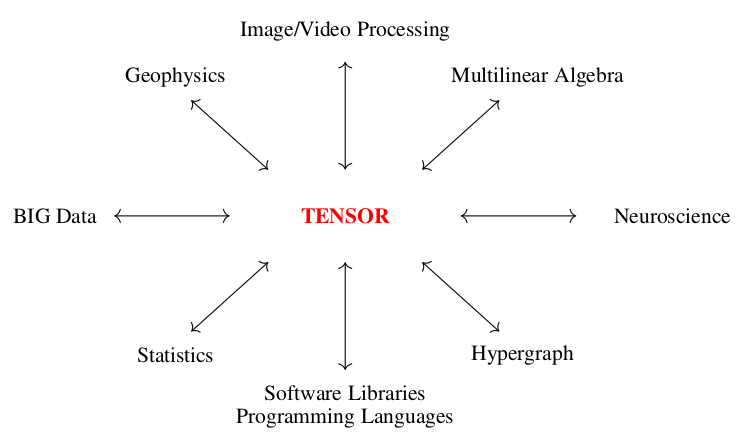### Home

#### Numerical Algorithms and Tensor Computing Laboratory

Numerical Algorithms and Tensor Computing Laboratory

#### Dr. Ratikanta Behera

Dr. Ratikanta Behera

# Welcome to NATC Lab

Welcome to the Numerical Algorithms and Tensor Computing Lab at the Indian Institute of Science, Bangalore. We are a part of Department of Computational and Data Sciences (CDS). The research lab is established in May 2022 with Ratikanta Behera as the convenor.

In Numerical Algorithms and Tensor Computing Lab, we are interested in the field of scientific computing with particular emphasis on the adaptive wavelets methods, numerical multilinear algebra, and neural networks.

Tensor computations are at the core of a multitude of applications in diverse domains of scientific computing and data science. These computations present several challenges due to their complexity, high computational cost, and large memory footprint. In numerical multilinear algebra, we develop novel HPC-driven algorithms and theories for solving important existing and emerging multidimensional problems. Specifically, interested in developing fast tensor algorithms for solutions to multilinear systems, nonlinear optimization problems, low-rank approximation, generalized inverses of tensors, and solutions to partial differential equations in high dimensions problems. Further, we design adaptive recurrent tensor neural networks to solve time-varying problems. In particular, we aim to prove theoretically and numerically the behavior of adaptive recurrent tensor neural networks under various activation functions.

Mathematical modeling of problems in science and engineering typically involves solving partial differential equations. However, in many situations, the small spatial scales are highly localized, and thus the efficient and accurate solution of the problem requires a locally adapted grid. Traditional adaptive algorithms are costly because grids can change drastically within a short time interval. Thus, conventional algorithms on a uniform grid are inefficient for astrophysics, material sciences, meteorology, combustion problems, and turbulence modeling. Our goal is to develop a dynamically adaptive wavelet collocation algorithm to deal with problems with localized structures, which might occur intermittently anywhere in the computational domain or change their locations and scales in space and time. We propose HPC-centric adaptive wavelet algorithms for solving partial differential equations and integral equations, data compression, signal recognition, and signal & image processing.

Our lab accommodates the studies undertaken on the topics related to but not limited to:

• High-performance tensor contractions in scientific computing

• Tensor generalized inverses and applications

• Tensor decompositions and approximations

• High-performance algorithms and implementations of tensor operations

• Optimization of algorithms for tensor computations

• Adaptive space-time wavelet algorithm for PDEs

• Design adaptive space-time curvelets and its applications

• Dynamic adaptive algorithm for image encryption

• Tensor methods in applied computational domains

• Tensor operations with application to Machine and Deep learning